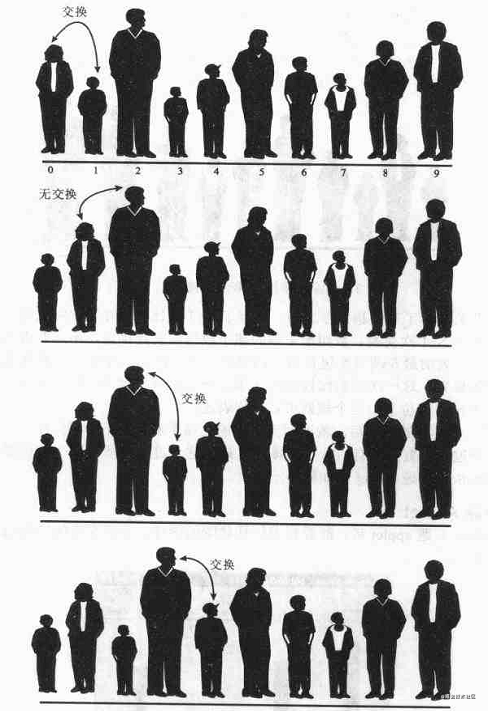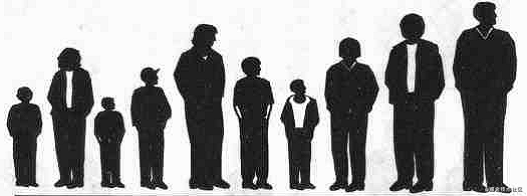# 排序算法之冒泡排序xcbeyond

# 2、实例分析# 4、算法代码：（Java 版）

/** * 冒泡排序 算法 * @author xcbeyond * */public class BubbleSort {	public static void main(String[] args) {		// TODO Auto-generated method stub		int a[] ={13,15,37,89,60,39,12,109,56,72} ;		int i = 0;		int j = 0;				for(i=0;i<10;i++)			System.out.print(a[i]+"  ");		System.out.println();				for(i=0;i<a.length;i++)			for(j=0;j<a.length-i-1;j++)			{				if(a[j]>a[j+1])				{					int temp;					temp=a[j];					a[j]=a[j+1];					a[j+1]=temp;				}			}				for(i=0;i<10;i++)			System.out.print(a[i]+"  ");			}}## 评论